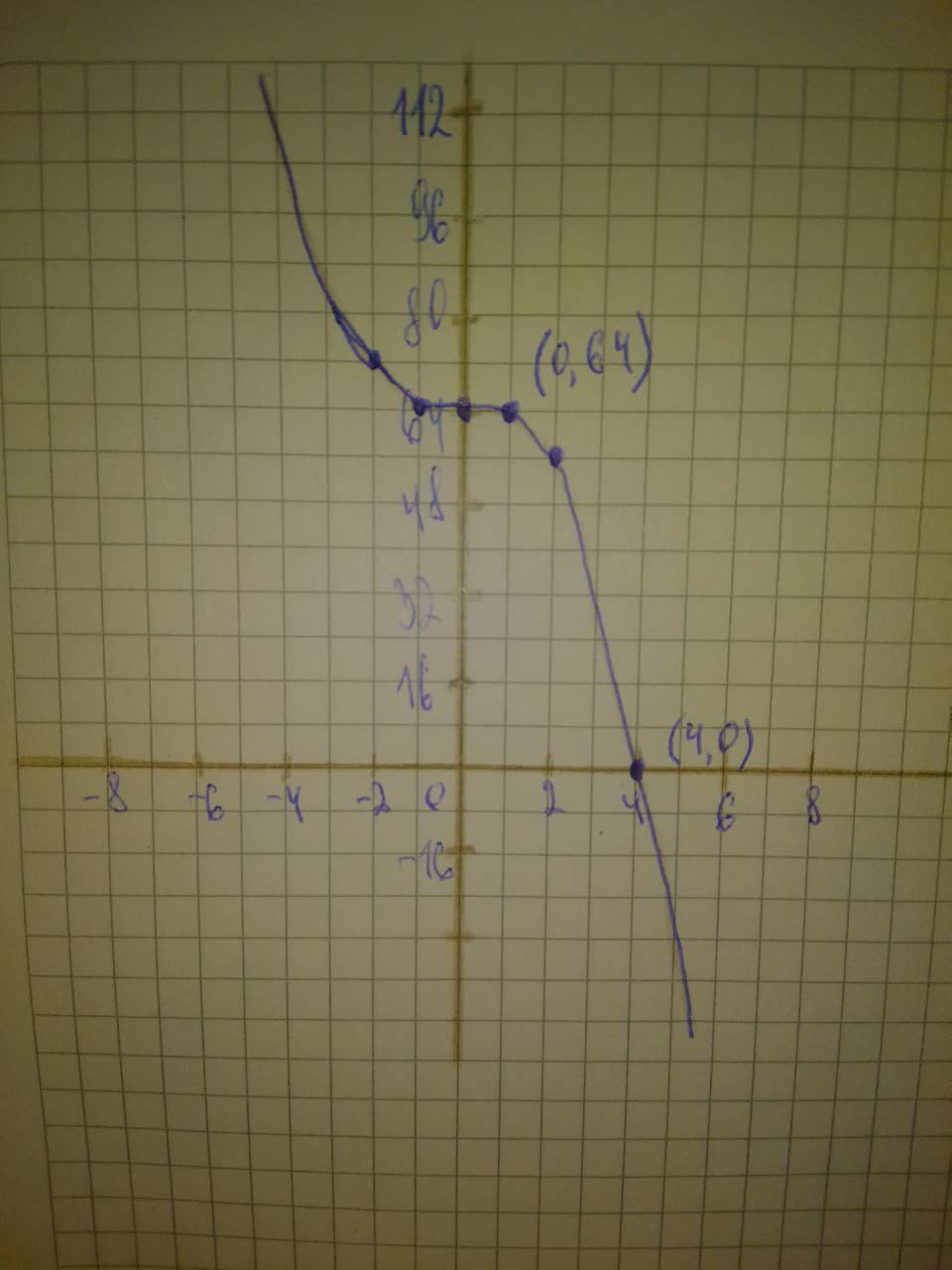Graph the polynomial by transforming an appropriate graph of thepermaneceerc 2021-08-13 Answered
Graph the polynomial by transforming an appropriate graph of the form $$\displaystyle{y}={x}^{{{n}}}$$.
Show clearly all x- and y-intercepts. $$\displaystyle{P}{\left({x}\right)}=-{x}^{{{3}}}+{64}$$

• Questions are typically answered in as fast as 30 minutes

Solve your problem for the price of one coffee

• Math expert for every subject
• Pay only if we can solve itcoffentw

Step 1
The parent function of $$\displaystyle{P}{\left({x}\right)}=-{x}^{{{3}}}+{64}\ {i}{s}\ {y}={x}^{{{3}}}$$, which passes through the points $$\displaystyle{\left(-{2},-{8}\right)},{\left(-{1},-{1}\right)},{\left({0},{0}\right)},{\left({1},{1}\right)}{\quad\text{and}\quad}{\left({2},{8}\right)}$$.
$$\displaystyle{P}{\left({x}\right)}=-{x}^{{{3}}}+{64}$$ is the graph of $$\displaystyle{y}={x}^{{{3}}}$$ reflected across the x-axis and then translated up 64 units. Reflecting the points on $$\displaystyle{y}={x}^{{{3}}}$$ across the x-axis gives (-2,8),(-1,1),(0,0),(1,-1) and (2,-8). Translating these points up 64 units then gives the points (-2,72), (-1,65),(0,64),(1,63), and (2,56).
The x-intercept of P(x) is when $$\displaystyle{P}{\left({x}\right)}={0}$$:
$$\displaystyle{P}{\left({x}\right)}=-{x}^{{{3}}}+{64}$$ Given function.
$$\displaystyle{0}=-{x}^{{{3}}}+{64}$$ Substitute $$\displaystyle{P}{\left({x}\right)}={0}$$
$$\displaystyle{x}^{{{3}}}={64}$$ Add $$\displaystyle{x}^{{{3}}}$$ on both sides.
$$\displaystyle{x}=\sqrt{{{3}}}{\left\lbrace{64}\right\rbrace}$$ Cube root both sides.
$$\displaystyle{x}={4}$$ Simplify
The x-intercept of P(x) is then the point (4,0). The y-intercept is when $$\displaystyle{x}={0}$$ which we already know is at the point (0,64). Plot the points, including the x-intercept, and then connect them with a smooth curve. Label the coordinates of the intercepts in your graph: